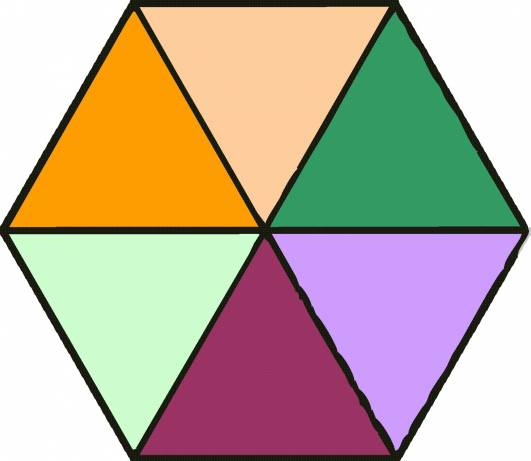# How To Calculate The Area Of A Hexagon?

There are three ways to calculate the area of a Hexagon. The easiest way of understanding the formula for this is to recognise that a regular hexagon is made up of six equilateral triangles. So the formula is evolved from the formula to find the area of an equilateral triangle.The first formula for finding the area of a hexagon is Area
= (3√3 s
2)/ 2 where 's' is the length of a side of the regular hexagon. You must identify the length of the side and substitute 's' with this number. If the value of 's' is 9cm for example your stage-by-stage working for the equation would be:

(3√3 x 92)/2 = (3√3 x
81)/2 = (243√3)/2 = 420.8/2 = 210.4 cm2

Other formulas include: Area = 1/2 x perimeter x apothem

Apothem = a line from the centre of a regular polygon at right
angles to any of its side

Perimeter = the continuous line forming the boundary of a closed
geometrical figure.

Area = The expanse within a shape.

If the perimeter is 60cm and the apothem is 5√3 your workings out would be:

◦  1/2 x 60 cm x 5√3 cm =

◦  30 x 5√3 cm =

◦  150√3 cm =

◦  259. 8 cm2

Here is way of calculating an Irregular Hexagon:

If you are given the vertices of the Hexagon you should create a chart with two columns and seven rows, each row being labelled by the six points and each column should be labelled with x or y of the points. Repeat the coordinates of the first point at the bottom of the list.

Vertices = each angular point of a polygon, polyhedron, or other figure.

Then multiply the x coordinates of each point by the next y coordinate and then add the results.

Then multiply the y coordinates of each point by the next x coordinate and then add the results.

Then subtract the second set of coordinates number by the first set of coordinates.

Then divide this difference by two.

thanked the writer.